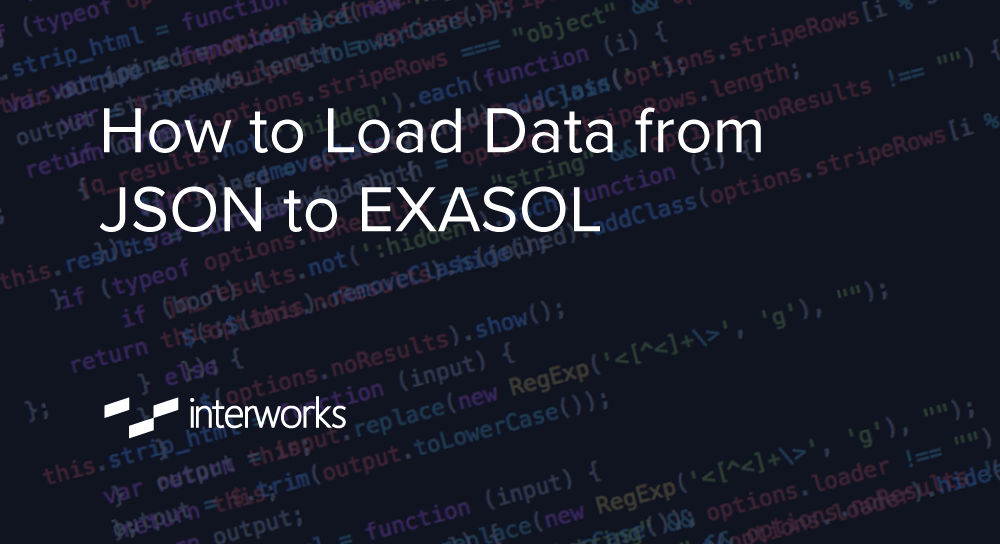×

Data

# How to Load Data from JSON to EXASOL//

If you’ve been looking for a way to load JSON data into EXASOL with just a click, then you’ll love this post! As data scientists, most of our time is spent preparing data for analysis and modeling. In my professional experience, I have faced many hurdles in getting the right data in the right format for analysis.

One common problem users find is scraping data from the web and storing it in a table or CSV. This post could be very useful for professionals and students alike who deal with data in a JSON format and would want to convert it to a tabular form. All you have to do is specify the URL that contains the data and this Python script will automatically normalize the data. The tabular data is then stored in EXASOL by creating the appropriate table.

## Normalizing the Data

The main step is to normalize the data structure that we retrieved from the URL. It could be in many formats such as a dictionary, list, nested lists and dictionaries:

```def json_db(url, dbinfo, table,db):
import pandas as pd
from pandas.io.json import json_normalize
import requests
import csv
from sqlalchemy import create_engine

# to retrieve data from url
r = requests.get(url)
r.text
data=r.json()```

The “json_normalize” function can be used if the data does not contain any nested items. Flattening objects with nested dictionaries and lists is not trivial. We must recursively extract values out of the object to create a flattened object. The flattened object is made as a pandas.DataFrame using “json_normalize” or “pd.DataFrame.” The function below is responsible for normalizing the data if the parent node is a dictionary:

```def flatten_dict(y):

out = {}

def smooth(x, name1='j'):

if type(x) is dict:

for a in x:

smooth(x[a], name1 + a + '_')

elif type(x) is list:

i = 0

for a in x:

smooth(a, name1 + str(i) + '_')

i += 1

else:

out[name1[:-1]] = x

smooth(y)

return out```

Please note that I am adding a prefix for every column name, because if the data contains any EXASOL key words such as a name or timestamp, then the table fails. The function below is responsible for normalizing the data if the parent node is a list:

```def flatten_list(data):

flat = []

def smooth(y):

out = {}

def flatten(x, name1='j'):

if type(x) is dict:

for a in x:

flatten(x[a], name1 + a + '_')

elif type(x) is list:

for a in x:

flatten(a, name1 + '_')

else:

out[name1[:-1]] = x

flatten(y)

return out

#Loop needed to flatten multiple objects
for i in range(len(data)):
flat.append(smooth(data[i]).copy())

return json_normalize(flat)```

After normalizization, we must call “pd.DataFrame” to make it a tabular form. Once we have the data in a tabular format, we can store it in any database that we want. However, EXASOL is known for its fast performance when it comes to handling large datasets and integrations. The function below creates a table automatically in EXASOL based on the columns from pandas.DataFrame. The script creates a table dynamically eliminating the need to change DML statements for every JSON structure.

For dynamic creation of tables and easier iteration, I am storing the pandas.DataFrame in a CSV file locally using “df.to_csv.” The values and column names are read from the CSV file. You can also skip this part and read directly from the pandas.DataFrame. I’m creating an insert operation placeholder by reading the columns. DML statements are then executed by enabling the connection using “create_engine” as you can see below:

```    def json_database(df,dbinfo,table,db):
if db is 'Exasol':
try:
engine=create_engine(dbinfo)
sql='''CREATE TABLE %s (x VARCHAR(10))''' % table
engine.execute(sql)
df.to_csv('out.csv', index=False)
f=open('out.csv','r')
ncol=len(col_name)
for j in range(ncol):
engine.execute('''ALTER TABLE %s DROP COLUMN x''' % table)
placeholders=(ncol-1) * "?, " + "?"
sql_insert='''INSERT INTO %s values (%s)''' % (table,placeholders)
engine.execute(sql_insert,rows)
return "Data added successfully in EXASOL!"```

If a table is already present in EXASOL, it will automatically enter the values in the table:

```except:
df.to_csv('out.csv', index=False)
f=open('out.csv','r')
ncol=len(col_name)
placeholders=(ncol-1) * "?, " + "?"
sql_insert='''INSERT INTO %s values (%s)''' % (table,placeholders)
engine.execute(sql_insert,rows)
return "Data added successfully in EXASOL!"```

## Calling the Sub-Functions

I’m calling normalized functions first based on the type of parent node in JSON. Next, I’ll make it a tabular form by calling pandas after the database operations are performed:

```if type(data) is dict:
flat = flatten_dict(data)
df=pd.DataFrame(flat,index=)
json_database(df,dbinfo,table,db)

if type(data) is list:
flat = flatten_list(data)
df=pd.DataFrame(flat)
json_database(df,dbinfo,table,db)

## Calling the Main Function

Connect to EXASOL using pyodbc and specifying the URL:

`json_db('url','exa+pyodbc://username:password@datasourcename/schemaname','tablename','EXASOL')`

This code can be easily extended to load data from JSON to other standard databases such as MySQL and PostgreSQL:

```def json_database(df,dbinfo,table,db):
if db is 'Mysql':
engine=create_engine(dbinfo)
df.to_sql(name=table, con=engine, if_exists='append', index=False)
return "Data added successfully in MySQL!"

if db is 'Postgresql':
engine=create_engine(dbinfo)
df.to_sql(name=table, con=engine, if_exists='append', index=False)
return "Data added successfully in PostgreSQL!"```

If you would like to connect to EXASOL and explore all its functionalities, contact us today! You can also find other great posts on EXASOL here.

### The Latest

• ##### Tableau Cloud System (SCIM): Unifying the Dimensions

How to Load Data from JSON to EXASOL If you’ve been looking for a way to load JSON data into EXASOL with just a click, then you’ll love this post! As data scientists, most ...
EXASOL – Small Business Edition Many of you must have heard about EXASOL. If not, I hope this blog gives you the motivation to discover it’s capabilities for yourself. ...

See more from this author →

InterWorks uses cookies to allow us to better understand how the site is used. By continuing to use this site, you consent to this policy. Review Policy OK

×

Interworks GmbH
Ratinger Straße 9
40213 Düsseldorf
Germany
Geschäftsführer: Mel Stephenson

Kontaktaufnahme: markus@interworks.eu
Telefon: +49 (0)211 5408 5301

Amtsgericht Düsseldorf HRB 79752
UstldNr: DE 313 353 072